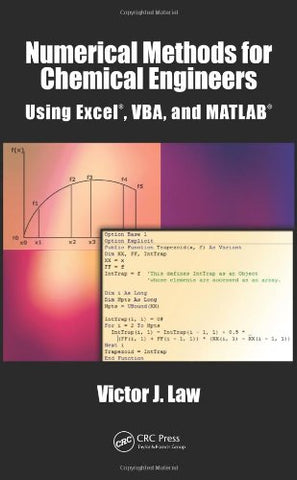# Numerical Methods for Chemical Engineers Using Excel, VBA, and MATLAB

• ISBN-13: 9781466575349
• \$105.82

Only 3 left!

While teaching the Numerical Methods for Engineers course over the last 15 years, the author found a need for a new textbook, one that was less elementary, provided applications and problems better suited for chemical engineers, and contained instruction in Visual BasicÂ® for Applications (VBA). This led to six years of developing teaching notes that have been enhanced to create the current textbook, Numerical Methods for Chemical Engineers Using ExcelÂ®, VBA, and MATLABÂ®. Focusing on Excel gives the advantage of it being generally available, since it is present on every computerâPC and Macâthat has Microsoft Office installed. The VBA programming environment comes with Excel and greatly enhances the capabilities of Excel spreadsheets. While there is no perfect programming system, teaching this combination offers knowledge in a widely available program that is commonly used (Excel) as well as a popular academic software package (MATLAB). Chapters cover nonlinear equations, Visual Basic, linear algebra, ordinary differential equations, regression analysis, partial differential equations, and mathematical programming methods.Each chapter contains examples that show in detail how a particular numerical method or programming methodology can be implemented in Excel and/or VBA (or MATLAB in chapter 10). Most of the examples and problems presented in the text are related to chemical and biomolecular engineering and cover a broad range of application areas including thermodynamics, fluid flow, heat transfer, mass transfer, reaction kinetics, reactor design, process design, and process control. The chapters feature "Did You Know" boxes, used to remind readers of Excel features. They also contain end-of-chapter exercises, with solutions provided.